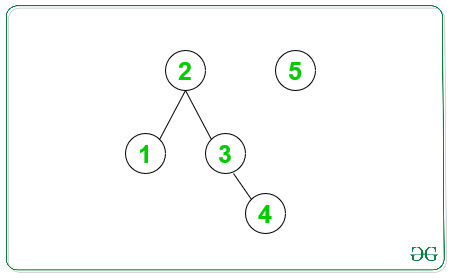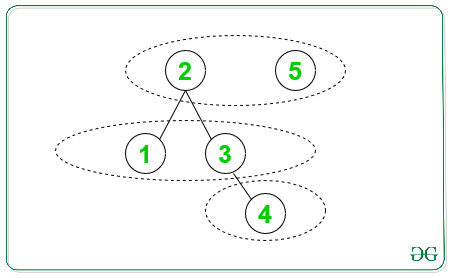# Minimum Bipartite Groups

• Difficulty Level : Expert
• Last Updated : 13 Aug, 2021

Given Adjacency List representation of graph of N vertices from 1 to N, the task is to count the minimum bipartite groups of the given graph.

Examples:

Input: N = 5
Below is the given graph with number of nodes is 5:Output:
Explanation:
Possible groups satisfying the Bipartite property: [2, 5], [1, 3], 
Below is the number of bipartite groups can be formed:Approach:
The idea is to find the maximum height of all the Connected Components in the given graph of N nodes to find the minimum bipartite groups. Below are the steps:

1. For all the non-visited vertex in the given graph, find the height of the current Connected Components starting from the current vertex.
2. Start DFS Traversal to find the height of all the Connected Components.
3. The maximum of the heights calculated for all the Connected Components gives the minimum bipartite groups required.

Below is the implementation of the above approach:

## C++

 `#include ``using` `namespace` `std;` `// Function to find the height sizeof``// the current component with vertex s``int` `height(``int` `s, vector<``int``> adj[],``           ``int``* visited)``{``    ``// Visit the current Node``    ``visited[s] = 1;``    ``int` `h = 0;` `    ``// Call DFS recursively to find the``    ``// maximum height of current CC``    ``for` `(``auto``& child : adj[s]) {` `        ``// If the node is not visited``        ``// then the height recursively``        ``// for next element``        ``if` `(visited[child] == 0) {``            ``h = max(h, 1 + height(child, adj,``                                  ``visited));``        ``}``    ``}``    ``return` `h;``}` `// Function to find the minimum Groups``int` `minimumGroups(vector<``int``> adj[], ``int` `N)``{``    ``// Initialise with visited array``    ``int` `visited[N + 1] = { 0 };` `    ``// To find the minimum groups``    ``int` `groups = INT_MIN;` `    ``// Traverse all the non visited Node``    ``// and calculate the height of the``    ``// tree with current node as a head``    ``for` `(``int` `i = 1; i <= N; i++) {` `        ``// If the current is not visited``        ``// therefore, we get another CC``        ``if` `(visited[i] == 0) {``            ``int` `comHeight;``            ``comHeight = height(i, adj, visited);``            ``groups = max(groups, comHeight);``        ``}``    ``}` `    ``// Return the minimum bipartite matching``    ``return` `groups;``}` `// Function that adds the current edges``// in the given graph``void` `addEdge(vector<``int``> adj[], ``int` `u, ``int` `v)``{``    ``adj[u].push_back(v);``    ``adj[v].push_back(u);``}` `// Drivers Code``int` `main()``{``    ``int` `N = 5;` `    ``// Adjacency List``    ``vector<``int``> adj[N + 1];` `    ``// Adding edges to List``    ``addEdge(adj, 1, 2);``    ``addEdge(adj, 3, 2);``    ``addEdge(adj, 4, 3);` `    ``cout << minimumGroups(adj, N);``}`

## Java

 `import` `java.util.*;` `class` `GFG{`` ` `// Function to find the height sizeof``// the current component with vertex s``static` `int` `height(``int` `s, Vector adj[],``           ``int` `[]visited)``{``    ``// Visit the current Node``    ``visited[s] = ``1``;``    ``int` `h = ``0``;`` ` `    ``// Call DFS recursively to find the``    ``// maximum height of current CC``    ``for` `(``int`  `child : adj[s]) {`` ` `        ``// If the node is not visited``        ``// then the height recursively``        ``// for next element``        ``if` `(visited[child] == ``0``) {``            ``h = Math.max(h, ``1` `+ height(child, adj,``                                  ``visited));``        ``}``    ``}``    ``return` `h;``}`` ` `// Function to find the minimum Groups``static` `int` `minimumGroups(Vector adj[], ``int` `N)``{``    ``// Initialise with visited array``    ``int` `[]visited= ``new` `int``[N + ``1``];`` ` `    ``// To find the minimum groups``    ``int` `groups = Integer.MIN_VALUE;`` ` `    ``// Traverse all the non visited Node``    ``// and calculate the height of the``    ``// tree with current node as a head``    ``for` `(``int` `i = ``1``; i <= N; i++) {`` ` `        ``// If the current is not visited``        ``// therefore, we get another CC``        ``if` `(visited[i] == ``0``) {``            ``int` `comHeight;``            ``comHeight = height(i, adj, visited);``            ``groups = Math.max(groups, comHeight);``        ``}``    ``}`` ` `    ``// Return the minimum bipartite matching``    ``return` `groups;``}`` ` `// Function that adds the current edges``// in the given graph``static` `void` `addEdge(Vector adj[], ``int` `u, ``int` `v)``{``    ``adj[u].add(v);``    ``adj[v].add(u);``}`` ` `// Drivers Code``public` `static` `void` `main(String[] args)``{``    ``int` `N = ``5``;`` ` `    ``// Adjacency List``    ``Vector []adj = ``new` `Vector[N + ``1``];``    ``for` `(``int` `i = ``0` `; i < N + ``1``; i++)``        ``adj[i] = ``new` `Vector();``    ` `    ``// Adding edges to List``    ``addEdge(adj, ``1``, ``2``);``    ``addEdge(adj, ``3``, ``2``);``    ``addEdge(adj, ``4``, ``3``);`` ` `    ``System.out.print(minimumGroups(adj, N));``}``}` `// This code is contributed by 29AjayKumar`

## Python3

 `import` `sys` `# Function to find the height sizeof``# the current component with vertex s``def` `height(s, adj, visited):` `    ``# Visit the current Node``    ``visited[s] ``=` `1``    ``h ``=` `0`` ` `    ``# Call DFS recursively to find the``    ``# maximum height of current CC``    ``for` `child ``in` `adj[s]:`` ` `        ``# If the node is not visited``        ``# then the height recursively``        ``# for next element``        ``if` `(visited[child] ``=``=` `0``):``            ``h ``=` `max``(h, ``1` `+` `height(child, adj,``                                  ``visited))``    ` `    ``return` `h` `# Function to find the minimum Groups``def` `minimumGroups(adj, N):` `    ``# Initialise with visited array``    ``visited ``=` `[``0` `for` `i ``in` `range``(N ``+` `1``)]`` ` `    ``# To find the minimum groups``    ``groups ``=` `-``sys.maxsize`` ` `    ``# Traverse all the non visited Node``    ``# and calculate the height of the``    ``# tree with current node as a head``    ``for` `i ``in` `range``(``1``, N ``+` `1``):`` ` `        ``# If the current is not visited``        ``# therefore, we get another CC``        ``if` `(visited[i] ``=``=` `0``):``            ``comHeight ``=` `height(i, adj, visited)``            ``groups ``=` `max``(groups, comHeight)``        ` `    ``# Return the minimum bipartite matching``    ``return` `groups` `# Function that adds the current edges``# in the given graph``def` `addEdge(adj, u, v):``    ` `    ``adj[u].append(v)``    ``adj[v].append(u)` `# Driver code   ``if` `__name__``=``=``"__main__"``:``    ` `    ``N ``=` `5`` ` `    ``# Adjacency List``    ``adj ``=` `[[] ``for` `i ``in` `range``(N ``+` `1``)]`` ` `    ``# Adding edges to List``    ``addEdge(adj, ``1``, ``2``)``    ``addEdge(adj, ``3``, ``2``)``    ``addEdge(adj, ``4``, ``3``)`` ` `    ``print``(minimumGroups(adj, N))` `# This code is contributed by rutvik_56`

## C#

 `using` `System;``using` `System.Collections.Generic;` `class` `GFG{``  ` `// Function to find the height sizeof``// the current component with vertex s``static` `int` `height(``int` `s, List<``int``> []adj,``           ``int` `[]visited)``{``    ``// Visit the current Node``    ``visited[s] = 1;``    ``int` `h = 0;``  ` `    ``// Call DFS recursively to find the``    ``// maximum height of current CC``    ``foreach` `(``int`  `child ``in` `adj[s]) {``  ` `        ``// If the node is not visited``        ``// then the height recursively``        ``// for next element``        ``if` `(visited[child] == 0) {``            ``h = Math.Max(h, 1 + height(child, adj,``                                  ``visited));``        ``}``    ``}``    ``return` `h;``}``  ` `// Function to find the minimum Groups``static` `int` `minimumGroups(List<``int``> []adj, ``int` `N)``{``    ``// Initialise with visited array``    ``int` `[]visited= ``new` `int``[N + 1];``  ` `    ``// To find the minimum groups``    ``int` `groups = ``int``.MinValue;``  ` `    ``// Traverse all the non visited Node``    ``// and calculate the height of the``    ``// tree with current node as a head``    ``for` `(``int` `i = 1; i <= N; i++) {``  ` `        ``// If the current is not visited``        ``// therefore, we get another CC``        ``if` `(visited[i] == 0) {``            ``int` `comHeight;``            ``comHeight = height(i, adj, visited);``            ``groups = Math.Max(groups, comHeight);``        ``}``    ``}``  ` `    ``// Return the minimum bipartite matching``    ``return` `groups;``}``  ` `// Function that adds the current edges``// in the given graph``static` `void` `addEdge(List<``int``> []adj, ``int` `u, ``int` `v)``{``    ``adj[u].Add(v);``    ``adj[v].Add(u);``}``  ` `// Drivers Code``public` `static` `void` `Main(String[] args)``{``    ``int` `N = 5;``  ` `    ``// Adjacency List``    ``List<``int``> []adj = ``new` `List<``int``>[N + 1];``    ``for` `(``int` `i = 0 ; i < N + 1; i++)``        ``adj[i] = ``new` `List<``int``>();``     ` `    ``// Adding edges to List``    ``addEdge(adj, 1, 2);``    ``addEdge(adj, 3, 2);``    ``addEdge(adj, 4, 3);``  ` `    ``Console.Write(minimumGroups(adj, N));``}``}`` ` `// This code is contributed by Rajput-Ji`

## Javascript

 ``

Output:

`3`

Time Complexity: O(V+E), where V is the number of vertices and E is the set of edges.
Auxiliary Space: O(V).

My Personal Notes arrow_drop_up